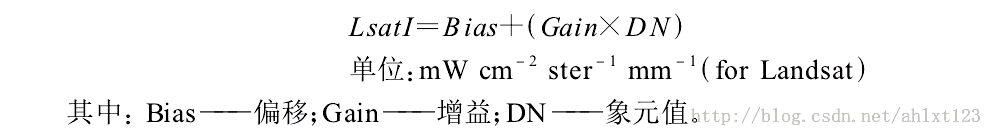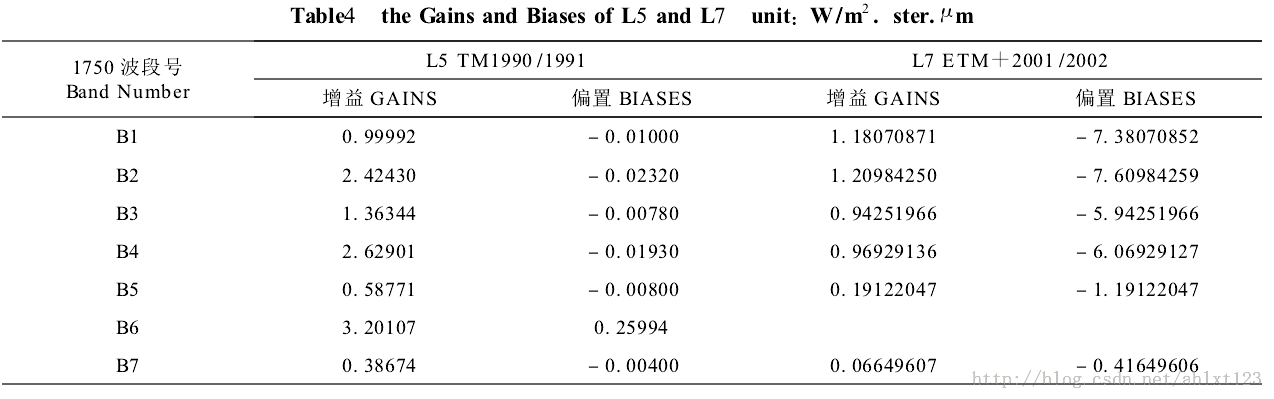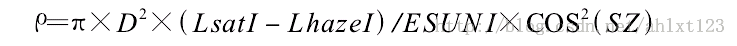# Python中使用OpenCV库来进行简单的气象学遥感影像计算

piaodoo 编程教程 2020-02-02 12:34:02 234 0 python教程

OpenCV的全称是Open Source Computer Vision Library，是一个跨平台的计算机视觉库。OpenCV是由英特尔公司发起并参与开发，以BSD许可证授权发行，可以在商业和研究领域中免费使用。OpenCV可用于开发实时的图像处理、计算机视觉以及模式识别程序。该程序库也可以使用英特尔公司的IPP进行加速处理。
OpenCV用C++语言编写，它的主要接口也是C++语言，但是依然保留了大量的C语言接口。该库也有大量的Python, Java and MATLAB/OCTAVE (版本2.5)的接口。这些语言的API接口函数可以通过在线文档获得。现在也提供对于C#, Ch,Ruby的支持。

1、遥感影像的光谱辐射定标```def computL(gain,Dn,bias):
return (gain*Dn+bias)

```

2、遥感影像的大气校正```#ESUN
ESUNI71=196.9
#
Lmini=-6.2
Lmax=293.7
#
Qcal=1
Qmax=255
LIMIN=Lmini+(Qcal*(Lmax-Lmini)/Qmax)
LI=(0.01*ESUNI71*cos*cos)/(math.pi*D*D)
Lhazel=LIMIN-LI ```

3、计算遥感影像的反射率（JD为遥感成像的儒略日(Julian Day)，计算公式为：

```JD=K-32075+1461*(I+4800+(J-14)/12)/4+367*(J-2-(J-14)/12*12)/12-3*((I+4900+(J-14)/12)/100)/4
```

I、J、K分别为年、月、日

```import cv2
import numpy as np
import math

#图像格式转换
img10=cv2.cvtColor(img1,cv2.COLOR_BGR2GRAY)

#计算JD
I=2003
J=2
K=20
JD=K-32075+1461*(I+4800+ (J-14)/12)/4+367*(J-2-(J-14)/12*12)/12-3*((I+4900+(J-14)/12)/100)/4
#设置ESUNI值
ESUNI71=196.9
#计算日地距离D
D=1-0.01674*math.cos((0.9856*(JD-4)*math.pi/180))
#计算太阳天顶角

inter=(math.pi*D*D)/(ESUNI71*cos*cos)

#大气校正参数设置
Lmini=-6.2
Lmax=293.7
Qcal=1
Qmax=255
LIMIN=Lmini+(Qcal*(Lmax-Lmini)/Qmax)
LI=(0.01*ESUNI71*cos*cos)/(math.pi*D*D)
Lhazel=LIMIN-LI

def copy(img,new1):
new1= np.zeros(img.shape,dtype='uint16')
new1[:,:] = img[:,:]

def computL(gain,Dn,bias):
return (gain*Dn+bias)

if __name__ == '__main__':
print 'D=',D
print 'cosZS=',cos
print 'Lhazel=',Lhazel
#计算图像反射率
result=np.zeros(img.shape,dtype='uint16')
for i in range(0,img.shape(1)):
for j in range(0,img.shape(0)):
Lsat=computL(1.18070871,img10[i,j],-7.38070852)
result[i,j]=inter*(Lsat-Lhazel)*1000

#保存图像
cv2.imwrite("F:\\result.tif", result)
cv2.namedWindow("Image")
cv2.imshow("Image", result)
cv2.waitKey(0)
```